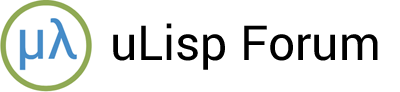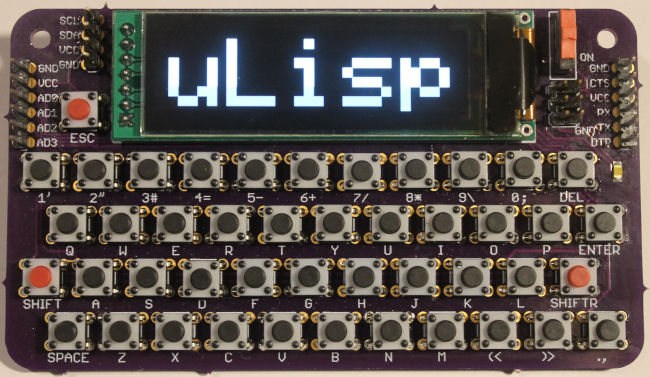# Big text mode

#1

Another idea I had as an addition for the Lisp Badge was a big-text mode, which would switch the display to double-sized characters, perhaps using a key combination to toggle between normal and big modes. This would have been useful for writing your name on the badge for use at conferences…

#2

I was able to implement this with plot3d and a small new built-in
function I named glyph-pixel.

### format function

Syntax: (glyph-pixel character x y)

The glyph-pixel function takes arguments character, x and y.
It returns a number representing the color value of the pixel at
offset (x y) within the glyph, in the current font, that
represents character. The offset’s origin (0 0) represents the
left-bottom pixel of character’s glyph. glyph-pixel will return
the nil color (typically 0) if the offset is outside the extents of
the glyph.

glyph-pixel adds 224 bytes to the program size. I pushed the changes to my sourcehut Lisp Badge repository. I’ll post a usage example separately.

#3

Here is an example that uses glyph-pixel to plot a string of glyphs at arbitrary scales and locations.

``````(defun plot-glyphs (m &optional sx sy tx ty)
"Plot the glyphs in string M.
Scale the glyph string by a factor of SX in the horizontal axis, and
SY in the vertical axis.  Translate the left bottom corner of the
glyph string to position (TX TY).  If none of the optional arguments
are specified, maximize the size of the glyph string and centre it in
the display."
(plot3d
(let* ((l (length m))
(mw (* l 6))
(mh (* 1 8))
(sx (or sx (/ 256 mw)))
(sy (or sy (/  64 mh)))
(gw (* (- mw 1) sx)) ;- 1 for trailing inter-character space
(gh (* (+ mh 1) sy)) ;+ 1 for leading inter-line space
(tx (or tx (ash (- 256 gw) -1))) ;tx nil centres horizontally
(ty (or ty (ash (-  64 gh) -1))) ;ty nil centres vertically
(pw (* 6 sx))) ;position width
(lambda (x y)
(let ((p (/ (- x tx) pw))) ;position
(if (or (< x tx) (>= x (+ tx gw))) 0
(glyph-pixel
(char m p)
(mod (/ (- x tx) sx) 6)
(/ (- y ty) sy))))))))
``````

Calling `(plot-glyphs "uLisp")` produces:#4

Nice!